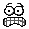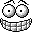# FUN for Figurenuts```	Each of the ten sets of equations below have nine blanks to be
filled in.  BUT, you must fill them in by using only the numbers 1 to 9
once and only once in each set.

Here is an example for Set 1:

(5 - 3) ÷ 2 = 1      6 + 4 - 9 = 1      (8 - 7) × 1 = 1

Now find another way for Set 1.  Then do the other sets in a
similar manner.

HAVE FUN!!!

Set 1:  (_ - _)  ÷ _ = 1		Set 2: (_ + _) ÷  _ = 2

_ + _   - _ = 1		 	_ + _  -  _ = 2

(_ - _)  × _ = 1		 	_ × _  -  _ = 2

Set 3:	(_ - _) ÷ _ = 3			Set 4:	(_ + _) ÷ _ = 4

(_ + _) ÷ _ = 3		 		 _ x _  ÷ _ = 4

_ - _  - _ = 3		 	 	 _ + _  - _ = 4

Set 5:	 _ ÷ _  + _ = 5			Set 6:	 _ ÷ _ ×  _ = 6

_ × _  - _ = 5		 		 _ + _ -  _ = 6

_ + _  - _ = 5		 		 _ - _ -  _ = 6

Set 7:	 _ ÷ _  + _ = 7			Set 8:	 _ ÷ _ + _  = 8

_ + _  - _ = 7		 		 _ × _ + _  = 8

_ × _  + _ = 7		 		 _ + _ - _  = 8

Set 9:	 _ ÷ _  + _ = 9			Set 10:	 _ ÷ _ + _  = 10

_ × _  + _ = 9		 		 _ + _ - _  = 10

_ × _  - _ = 9		 		 _ + _ - _  = 10

tt(8/25/84)

Source: Recreational Mathematics Magazine, Issue #1, February 1961, page 25.

```